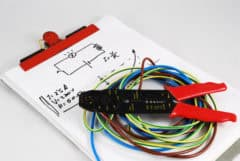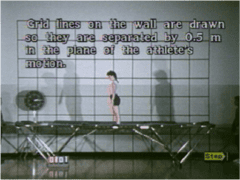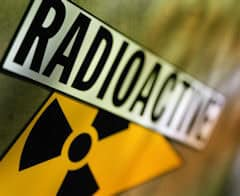## Electric circuitsIn this activity students use the current and voltage sensors and investigate power delivered to a resistor by investigating the behavior of bulbs in a dc circuit.

## Harmonic motionIn this activity students measure on the video clip and graph the movement of a trampoline jumper. Students create the velocity and acceleration versus time graphs and analyse both graphs. They investigate the relationship investigate the relationship between the position, the velocity and the acceleration of the jumper.

## Heat and temperatureIn this activity students record temperature during a cooling process of hot water and check the validity of Newton's law of cooling for the collected data

## Newton's laws of motionIn this activity students explore radioactive decay via a given decay model. Based on the model they determine the function, which describes the radioactive decay. Further they modify the model to describe a decay of a real radioactive isotope e.g. Barium 137m and compare how well the model fits the experimental results.In this activity students determine the speed of sound in air. There are two measuring methods offered in the activity. Depending on an interface a different method can be used